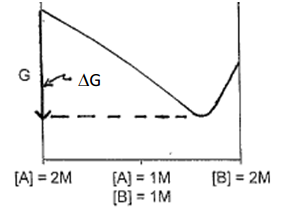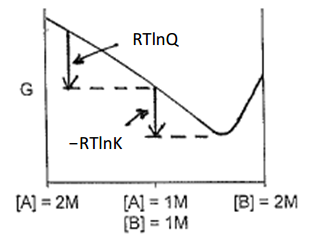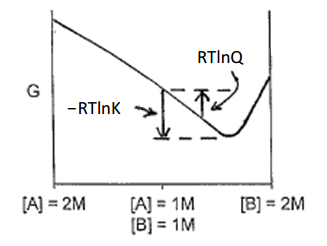# 4.1. Reactivity and The Standard State

Before examining chemical reactivity and activity it is useful to revisit chemical energy. The chemical energy of a system is known as the Gibbs energy and is denoted by G. Suppose we wanted to think about the simplest chemical reaction possible – a situation in which A reacts to produce B.

$\mathrm{A \Leftrightarrow B}$

A bottle of pure A has some amount of chemical energy. A bottle of pure B has some amount of chemical energy. A solution of A in water that is 2 Molar has some amount of chemical energy. A solution of B in water that is 2 Molar has some amount of chemical energy.

Do all four examples listed above (pure A, pure B, [A] = 2 M, B = [2 M]) have the same or different chemical energy?

Hopefully it will seem reasonable or intuitive to think that all four of these systems will have different chemical energies. Since the two compounds are different from each other, in their pure forms they likely have different chemical energies. Similarly, a chemical species dissolved in a solvent is now different than the pure compound and the two will have different chemical energies.

How would you measure or determine the absolute chemical energy of those four systems?

This is actually a trick question. We do not have methods available to determine the absolute chemical energy for a system. In reality the best that we can do is measure the difference in chemical energies between two systems. This difference is denoted as ΔG, which you have already studied in the preceding weeks and in other prior coursework.

Let’s continue to examine the situation in which A reacts to produce B.

$\mathrm{A \Leftrightarrow B}$

When will the chemical energy (G) of this system achieve its lowest value?

The lowest value of chemical energy for a reaction occurs when it is at equilibrium. For the example above, this means that pure A and pure B must have a higher chemical energy than the equilibrium state of the reaction. It is important to note that chemical systems strive to have the lowest chemical energy as that will be their most stable state.

We will next consider what a plot of G versus the mole fraction of A and B in which the situation

$\mathrm{[A] + [B] = 2\: Molar}$

is always satisfied. We can consider what the plot would look like for two different situations:

The reaction of A to produce B has a fairly large equilibrium constant.

The reaction of A to produce B has a fairly small equilibrium constant.

Remember that the equilibrium constant expression is:

$\mathrm{K= \dfrac{[B]}{[A]}}$

Since the equilibrium situation will have some concentration of both A and B, the system at equilibrium must have a lower chemical energy than either a 2 M solution of A or a 2M solution of B. That would allow us to draw two possible representations for the situation: one in which Keq is large (there is a much higher concentration of B than A), shown in the left panel of Figure 1; and one in which Keq is small (there is a much higher concentration of A than B), shown in the right panel of Figure 1.Figure 1. Plot of G versus molar quantities of reactant and product for a reaction with a large (left) and small (right) equilibrium constant (Keq).

Remember that we do not know how to calculate the true, absolute value of G. What we can do is quantify the difference in chemical energy (ΔG) between two different states (e.g., we could possibly measure the difference in chemical energy between a solution in which [A] = 2 M and equilibrium). The plot in Figure 2 illustrates the observed ΔG for such a situation (using the example of when Keq is large).Figure 2. Magnitude of ΔG between [A] = 2 M and equilibrium.

Why do we need to define something called the standard state?

Consider an example where every student in a class was asked to perform a laboratory measurement of ΔG for the reaction of A to produce B. If we consider the example where K is large, you could imagine a situation in which one student starts with a solution where the concentration of A is 2 M and B is 0 while another starts with a solution where the concentration of B is 2 M and A is 0. Looking at the plot in Figure 2, both students may measure accurate and correct values for ΔG but the values that the students report will be different. As seen by the plot in Figure 2, the value one would measure for ΔG is dependent on the particular starting point, since each starting point has a different initial value of G. In order to record ΔG values that can be tabulated and compared, everyone must agree in advance to a uniform set of starting conditions. These agreed upon starting conditions are known as the standard state.

What conditions constitute the standard state?

The standard state is the situation where every reactant and product in the reaction has an activity of 1. Since there are many conditions where we are unable to determine the exact activity of a substance, an approximation is made where the standard state has the concentration of all soluble species in the reaction at 1 Molar, gases at a pressure of 1 atmosphere and a temperature of 25oC (298 K). In addition, the standard state assumes that each of the soluble species behave as if they are the only compound present in the solution. This is called the assumption of a dilute solution, or an infinite dilution.

For A reacting to produce B, the standard state would be a solution in which [A] = 1 M and [B] = 1 M. The plot in Figure 3 shows the difference in chemical energy between the standard state and equilibrium for the situation in which Keq is large. The difference in chemical energy between the standard state and equilibrium is given a special designation: ΔGo.Figure 3. Difference in chemical energy between the standard state and equilibrium (ΔGo) for a reaction with a large equilibrium constant.

You can imagine that it might be difficult to think about how you could actually make a standard state solution and somehow hold it in a suspended state before allowing it to react and achieve equilibrium, thereby allowing a measurement of ΔGo. Meaning, it is difficult to prepare a solution of species A and species B that are both at 1 M concentration but in which both species behave as if they are the only compounds present in solution (the dilute solution, or infinite dilution assumption). [As an aside, electrochemical cells are actually ideal systems for measurements of ΔGo because it is possible to prepare the two half reactions in separate chambers but prevent the reaction from taking place by not completing all of the circuitry between the two chambers.]

What does it mean for the difference in chemical energy (ΔG or ΔGo) to be positive or negative?

In considering this, it is important to examine the two different plots of G for the reaction with a large Keq and small Keq. These are shown in Figure 4 and the value of ΔGo is indicated in each plot.Figure 4. ΔGo for a reaction with a large (left) and small (right) equilibrium constant.

Note that in both cases the chemical energy drops as the system goes from the standard state to equilibrium. However, when we think about carrying out a chemical reaction, our usual goal is to have the reaction form products. For this reason, we adopt a convention that says that ΔGo is less than 0 (negative) for a reaction that favors the formation of products. ΔGo is greater than 0 (positive) for a reaction that favors the formation of reactants.

The following equation is something you should have seen before in a unit on thermodynamics in either a general or physical chemistry course.

$\mathrm{∆G^o= -RT \ln K}$

This equation provides the difference in chemical energy between the standard state and equilibrium. Note that if Keq is large (favors products), lnKeq is positive and ΔGo is negative. Similarly if Keq is small (favors reactants), lnKeq is negative and ΔGo is positive.

Suppose the Starting Conditions are not at the Standard State

There are many situations where you do not want to examine reactions that begin with standard state conditions but instead want to start with a set of non-standard state conditions. The difference in chemical energy between non-standard state conditions and equilibrium is designated as ΔG. The equation that allows us to calculate ΔG is as follows:

$\mathrm{∆G= -RT\ln K + RT\ln Q\: or\: \Delta G= \Delta G^o + RT\ln Q}$

As seen previously, the term Q is an expression that looks exactly like the equilibrium constant expression except that it is evaluated using the starting non-standard state concentrations. It is important to recognize what each part of this equation is providing.

–RTLnKeq (denoted as Go) is the difference in chemical energy between the standard state and equilibrium.

RTlnQ is the difference in chemical energy between the non-standard state starting conditions and the standard state.

The plot in Figure 5 shows the placement of these two terms for the example of A reacting to produce B that we have been examining. In this case the non-standard state conditions are further removed from chemical equilibrium than the standard state. Note that with [A] = 1.50 M and [B] = 0.50 M, the value of Q is 0.33.

$\mathrm{Q= \dfrac{[B]}{[A]}= \dfrac{(0.50)}{(1.50)}=0.33}$

With the value of Q below 1, lnQ will be negative and the value of ΔG is more negative than the value of Go.Figure 5. Representation of RTlnQ and –RTlnK for a reaction starting in non-standard state conditions. Non-standard state conditions are further from equilibrium than the standard state conditions.

The plot in Figure 6 shows starting non-standard state conditions that are closer to equilibrium than those of the standard state: [A] = 0.50 M; [B] = 1.50 M. In this case the value of Q is 3.0.

$\mathrm{Q= \dfrac{[B]}{[A]}= \dfrac{(1.50)}{(0.50)}=3.0}$

With the value of Q above 1, lnQ is positive and the value of G is less negative than the value of Go.Figure 6. Representation of RTlnQ and –RTlnK for a reaction starting in non-standard state conditions. Non-standard state conditions are closer to equilibrium than the standard state conditions.